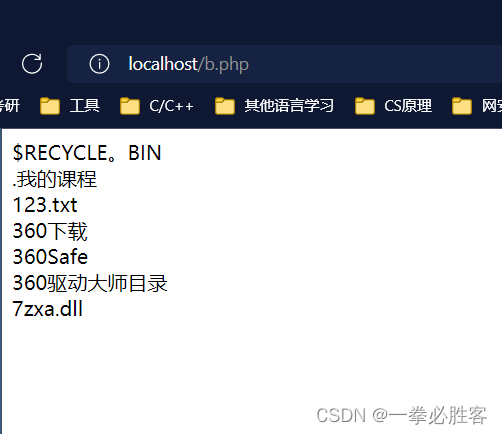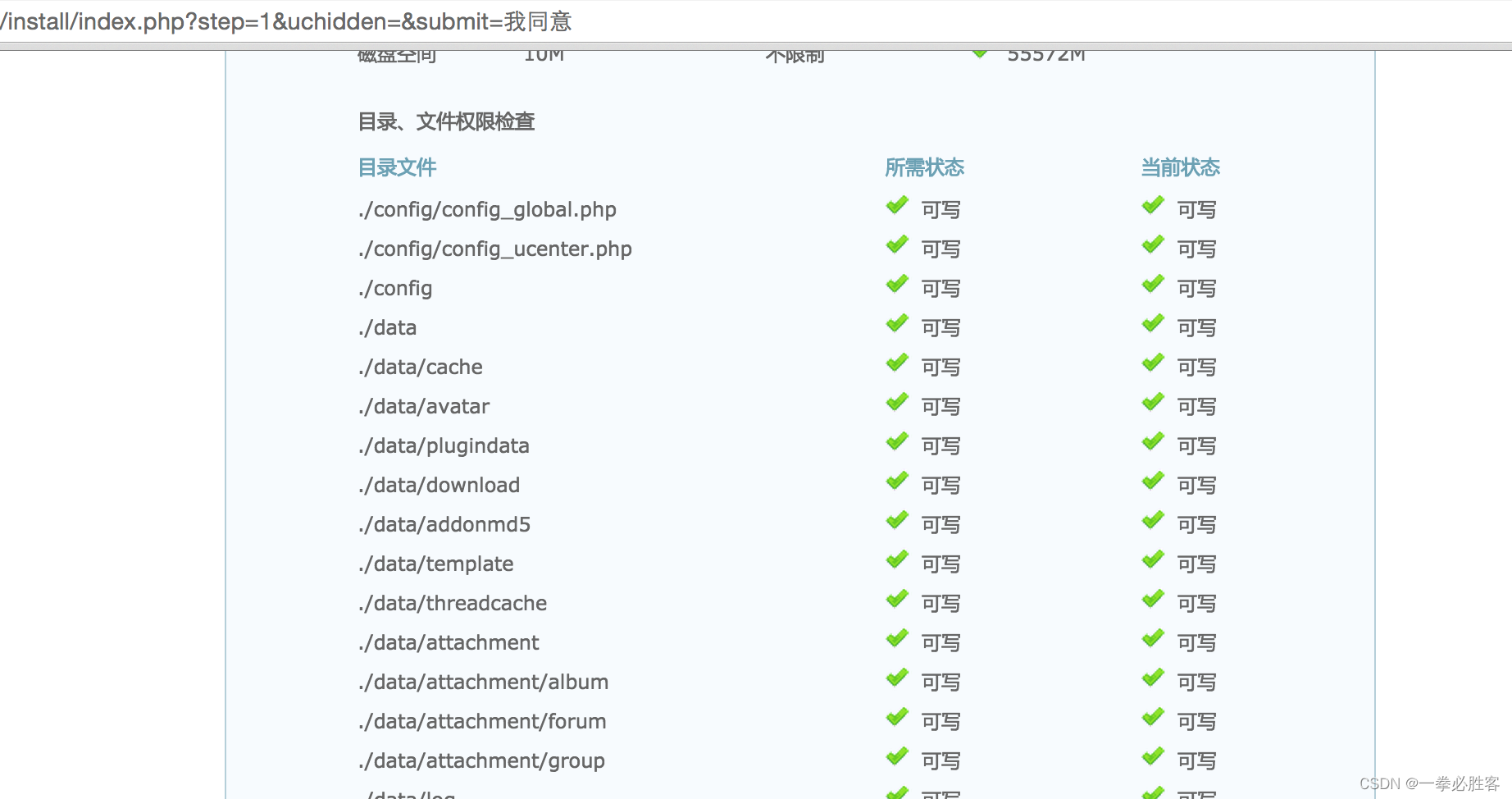# PHP系列（九）PHP文件系统处理

+关注继续查看

php文件系统处理

1、文件系统概述

is_dir( ) -判断给定文件名是否是一个目录

is_executable( ) -- 判断给定文件名是否可执行

is_file( ) -判断给定文件名是否为一个正常的文件

is_writable( ) -- 判断给定的文件名是否可写

2、文件的属性

//获取文件属性的函数

Function getFilePro($filename) { //文件是否存在 if(file_exists($filename)) {

echo"这个文件存在<br>";

//获取文件的类型

getFileType($filename); if(is_dir($filename)) {

echo"这是一个目录<br>";

}

if(is_file($filename)) { echo"这是一个文件<br>"; echo"文件大小为：".tosize(filesize($filename))."<br>";

}

//获取文件权限

if(is_readable($filename)) { echo"文件可读<br>"; } if(is_writable($filename)) {

echo"文件可写<br>";

}

if(is_executable($filename)) { echo"文件可执行<br>"; } //创建时间 echodate("Y-m-d H:i:s",filectime($filename))."<br>";

//访问时间

echodate("Y-m-d H:i:s",fileatime($filename))."<br>"; //修改时间 echodate("Y-m-d H:i:s",filemtime($filename))."<br>";

}else {

echo"这个文件不存在<br>";

}

}

//文件大小转换函数

functiontosize($size) {$s=$size;$dw="";

if($size> pow(2, 40)) {$s= $size/pow(2, 40);$dw= "TB";

}elseif($size > pow(2, 30)) {$s= $size/pow(2, 30);$dw= "GB";

}elseif($size > pow(2, 20)) {$s= $size/pow(2, 20);$dw= "MB";

}elseif($size > pow(2, 10)) {$s= $size/pow(2, 10);$dw= "KB";

}else{

$s=$size;

$dw= "types"; } return$s.$dw; } //获取文件的类型 functiongetFileType($filename){

switch(filetype($filename)) { case'dir': echo"这是一个目录<br>"; break; case'char': echo"这是一个字符设置<br>"; break; case'block': echo"这是一个块设备<br>"; break; case'file': echo"这是一个文件<br>"; break; case'link': echo"这是一个链接<br>"; break; default: echo"末知类型<br>"; } } getFilePro("./feng.txt"); 3、解析目录路径 DIRECTORY_SEPARATOR 代表 / PATH_SEPARATOR 代表 ; PHP_EOL 代表 换行符 4、遍历目录 //打开目录资源$dir = opendir("plus");

while($filename = readdir($dir)) {

//不要操作...

if($filename!="."&&$filename!="..") {

//一定要注意路径， 找对才可以

$filename ="./plus/".$filename;

if(is_dir($filename)) { echo "目录:".$filename."<br>";

} else {

echo "文件:".$filename."<br>"; } } } rewinddir($dir);//倒回目录句柄

echo"@@@@@@@@@@@@@<br>";

while($filename = readdir($dir)) {

//不要操作...

if($filename!="."&&$filename!="..") {

//一定要注意路径， 找对才可以

$filename ="./plus/".$filename;

if(is_dir($filename)) { echo "目录:".$filename."<br>";

} else {

echo "文件:".$filename."<br>"; } } } //关闭这个资源 closedir($dir);

//opendir()打开目录句柄

//is_dir()判断给定文件名是否是一个目录

//rewinddir()倒回目录句柄

//closedir()关闭目录句柄

//glod()遍历目录里文件

foreach(glob("member/*") as $filename ) { echo$filename."<br>";

}

5、统计目录的个数和大小

$total = disk_total_space("c:"); //统计磁盘总大小的$free = disk_free_space("c:");//统计磁盘可用空间大小

echo"C:盘约总大小：".round($total/pow(2,30))."G<br>"; echo"C:盘可用空间：".round($free/pow(2,30))."G<br>";

$dirn =0;//目录数$filen =0;//文件数

//用来统计一个目录下的文件和目录的个数

functiongetdirnum($file){ global$dirn;

global $filen;$dir = opendir($file); while($filename = readdir($dir)){ if($filename!="."&& $filename!=".."){$filename =$file."/".$filename;

if(is_dir($filename)){$dirn++;

getdirnum($filename);//递归、就可以查看所有子目录 }else{$filen++;

}

}

}

closedir($dir); } getdirnum("plus"); echo"目录数为：{$dirn}<br>";

echo"文件数为：{$filen}<br>"; //统计一个目录大小 functiondirsize($file){

$size = 0;$dir = opendir($file); while($filename = readdir($dir)){ if($filename!="."  && $filename!=".."){$filename =$file."/".$filename;

if(is_dir($filename)){ //使用递归$size +=dirsize($filename); }else{$size += filesize($filename); } } } closedir($dir);

return$size; } echo"目录大小为：".dirsize("member")/pow(1024,2)."Mb<br>"; 6、建立与删除目录 //mkdir("meizi"); 新建目录 //mkdir("finger",0644); //rmdir("meizi"); 删除目录 //rmdir("finger"); //unlink 删除文件 functiondeldir($dirname){

if(!file_exists($dirname)){ die("文件夹不存在！"); } if(is_file($dirname)){

unlink($dirname); }$dir = opendir($dirname); while($filename = readdir($dir)){ if($filename !="."&& $filename!=".."){$filename =$dirname."/".$filename;

if(is_dir($filename)){ deldir($filename);

}else{

unlink($filename); } } } } deldir("aaa"); 7、复制移动目录 //$dirsrc源目录

//$dirto 目标目录 function copydir($dirsrc, $dirto){ //如果原来的文件存在、是不是一个目录 if(file_exists($dirto)){

if(!is_dir($dirto)){ echo "目标不是一个目录、不能copy进去<br>"; exit; } }else{ mkdir($dirto);

}

$dir =opendir($dirsrc);

while($filename= readdir($dir)){

if($filename != "."&&$filename!=".."){

$srcfile =$dirsrc."/".$filename;//原文件$tofile =$dirto."/".$filename;//目标文件

if(is_dir($srcfile)){ copydir($srcfile,$tofile); //递归处理所有子目录 }else{ copy($srcfile, $tofile); } } } } copydir("member","E:/cisco"); 8、文件的一些基本操作函数 //创建一个空文件 //touch("./feng.txt"); //复制文件 //copy("feng.txt","meize.txt"); //移动或重新命名一个文件 //rename("meize.txt","meizi.txt"); //删除一个文件 //unlink("meizi.txt"); //$fp = fopen("feng.txt","w");

//      ftruncate($fp, 100); 把文件截断到指定的长度 //对文件内容的操作 // file_get_contents(""); //file_put_contents("meizi.txt","妹子今年18"); 写入文件内容 //file_put_contents("meizi.txt","小妹子今年19"); //echo file_get_contents("meizi.txt"); 读取文件内容 //file_put_contents("baidu.txt",file_get_contents("http://www.baidu.com")); //readfile("http://www.baidu.com");输出一个文件$arr =file("meizi.txt"); //file 把整个文件读入一个数组中

echocount($arr); echo'<br>'; echo$arr;

9、文件的打开与关闭

<?php

//使用绝对路径打开file.txt文件，选择只读模式，并返回资源$handle$handle =fopen("/home/rasmus/file.txt", "r");

//访问文档根目录下的文件，也以只读模式打开

$handle =fopen({$_SERVER['DOCUMENT_ROOT']}/data/info.txt","r");

// Windows 平台上，转义文件路径中的每个反斜线，或者用斜线，

$handle =fopen("c:\\data\\file.gif", "wb"); //使用相对路径打开file.txt文件，选择只读模式，并返回资源$handle

$handle =fopen("../data/info.txt", "r"); //打开远程文件， 使用HTTP协议只能以只读的模式打开$handle =fopen("http://www.example.com/", "r");

//使用FTP协议打开远程文件，如果FTP服务器可写，则可以以写的模式打开

$handle =fopen("ftp://user:password@example.com/somefile.txt", "w");10、文件内容操作 写入文件 fwrite -- 写入文件（可安全用于二进制文件） 语法：int fwrite ( resource handle,string string [, int length] ) fwrite() string 的内容写入 文件指针 handle 处。 如果指定 length，当写入了 length 个字节或者写完了 string 以后，写 入就会停止，视乎先碰到哪种情况。 返回写入的字符数，出现错误时则返回 FALSE 读取文件内容 函数名作用 fread( ) 读取文件（可安全用于二进制文件） file_get_contents() 将文件读入字符串 fgets()从打开的文件中读取一行 fgetc() 从打开的文件中读取一个字符 file()把文件读入一个数组中（无需使用fopen打开） readfile() 读取一个文件，并输出到输出缓冲（无需使用 fopen打开） 移动文件指针 ftell -- 返回文件指针读/写的位置 语法：int ftell ( resource handle ) 返回由 handle 指定的文件指针的位置，也就是文件流中的偏移量。 如果出错，返回 FALSE。文件指针必须是有效的，且必须指向一个通 fopen() popen()成功打开的文件。 fseek -- 在文件指针中定位 语法：int fseek ( resource handle,intoffset [,int whence] ) 在与 handle 关联的文件中设定文件指针位置。新位置，从文件头开始 以字节数度量，是以 whence 指定的位置加上 offsetwhence 的值定 义为： SEEK_SET - 设定位置等于 offset 字节。 SEEK_CUR - 设定位置为当前位置加上 offset SEEK_END - 设定位置为文件尾加上 offset。（要移动到文件尾之前 的位置，需要给 offset 传递一个负值。） 如果没有指定 whence，默认为 SEEK_SET 成功则返回 0；否则返回 -1。注意移动到 EOF 之后的位置不算错误 rewind -- 倒回文件指针的位置 语法：bool rewind ( resource handle ) handle 的文件位置指针设为文件流的开头。 如果成功则 返回 TRUE，失败则返回 FALSE 文件指针必须合法，并 且指向由 fopen() 成功打开的文件。 <?php //以只读模式打开文件$fp = fopen('data.txt', 'r') or die("文件打开失败");

echo ftell($fp)."<br>"; //输出刚打开文件指针默认位置，指针在文件开头位置为0 echo fread($fp, 10)."<br>";//读取文件中的前10个字符输出，指针位置发生了变化

echo ftell($fp)."<br>"; //读取文件前10个字符后，指针移动位置在第10个字节处 fseek($fp,100, SEEK_CUR); //将文件指针的位置，由当前位置向后移动100个字节数

echoftell($fp)."<br>"; //文件位置在110个字节处 echofread($fp, 10)."<br>"; //读取110120字节数位置的字串，读取后指针位置为120

fseek($fp,-10, SEEK_END); //又将指针移动到倒数10个字节位置处 echofread($fp, 10)."<br>"; //输出文件中最后10个字符

rewind($fp);//又移动文件指针到文件的开头 echoftell($fp)."<br>"; //指针在文件的开头位置，输出0

fclose($fp);//关闭文件资源 留言板 header("Content-Type:text/html;charset=utf8");$filename = "message.txt";

//如果用户提交了， 就写入文件， 按一定格式写入

if(isset($_POST['dosubmit'])){ //字段的分隔使用||, 行的分隔使用[n]$mess ="{$_POST['username']}||".time()."||{$_POST['title']}||{$_POST['content']}[n]"; writemessage($filename,$mess); } if(file_exists($filename)){

readmessage($filename); } function writemessage($filename, $mess){$fp = fopen($filename, "a"); fwrite($fp,$mess); fclose($fp);

}

function readmessage($filename) {$mess = file_get_contents($filename);$mess = rtrim($mess, "[n]");$arrmess = explode("[n]", $mess); foreach($arrmessas $m) { list($username, $dt,$title, $content) = explode("||",$m);

echo"<b>{$username}</b>, ".date("Y-m-dH:i").": <i>{$title}</i>,<u>{$content}</u><br><hr><br>"; } } ?> <formaction="message.php" method="post"> 用户: <input type="text"name="username" value="" /><br> 标题：<input type="text"name="title" value="" /><br> 内容：<textarea name="content" cols="40"rows="4"></textarea><br> <input type="submit"name="dosubmit" value="留言" /><br> </form> 11、文件的锁定机制 <?php header("Content-Type:text/html;charset=utf8");$filename = "message.txt";

//如果用户提交了， 就写入文件， 按一定格式写入

if(isset($_POST['dosubmit'])) { //字段的分隔使用||, 行的分隔使用[n]$mess ="{$_POST['username']}||".time()."||{$_POST['title']}||{$_POST['content']}[n]"; writemessage($filename,$mess); } if(file_exists($filename)) {

readmessage($filename); } function writemessage($filename, $mess){$fp = fopen($filename, "a"); if(flock($fp,LOCK_EX+LOCK_NB)) {//加锁

fwrite($fp,$mess);

flock($fp, LOCK_UN+LOCK_NB);//解锁 } else { echo "写入锁定失败!"; } fclose($fp);

}

function readmessage($filename) { //$mess= file_get_contents($filename);$mess = "";

$fp = fopen($filename, "r");

flock($fp,LOCK_SH+LOCK_NB); //读锁定 while(!feof($fp)) {

$mess.=fread($fp, 1024);

}

flock($fp,LOCK_UN+LOCK_NB); //释放$mess = rtrim($mess, "[n]");$arrmess = explode("[n]", $mess); foreach($arrmessas $m) { list($username, $dt ,$title, $content) = explode("||",$m);

echo"<b>{$username}</b>, ".date("Y-m-dH:i").": <i>{$title}</i>,<u>{$content}</u><br><hr><br>"; } fclose($fp);

}

?>

<formaction="message.php" method="post">

标题：<input type="text"name="title" value="" /><br>

内容：<textarea name="content" cols="40"rows="4"></textarea><br>

<input type="submit"name="dosubmit" value="留言" /><br>

</form>

12、文件上传设置

<html>

<body>

<inputtype="hidden" name="MAX_FILE_SIZE"value="1000000">

<inputtype="submit" value="上传文件">

</form>

</body>

</html>

POST方法: 表单最常用的功能,向目标页面传递变量,我们在上传文件的时候，会在表单中设置相应的属性，来完成文件的传递

enctype="multipart/form-data" 这样服务器就会知道，我们要传递一个文件,这样服务器可以知道上载的文件带有常规的表单信息。

MAX_FILE_SIZE 此字段必须在文件输入字段之前，控制最大的传递文件的大小(字节)

<input  type="file"name="userfile"> 设置浏览器文件输入浏览按钮

PHP配置文件中与文件上传有关的选项指令名默认值功能描述

$_FILES多维数组 超级全局数组$_FILES

1$_FILES["myfile"]["name"]中的值是: 客户端文件系统的文件的名称 2$_FILES["myfile"]["type"]中的值是: 客户端传递的文件的类型

3$_FILES["myfile"]["size"]中的值是: 文件的字节的大小 4$_FILES["myfile"]["tmp_name"]中的值是：– 文件被上传后在服务器存储的临时全路径

5$_FILES["myfile"]["error"]中的值是: 文件上传的错误代码－php 4.2以后增加的功能 13、文件上传的处理 在服务器端通过PHP处理上传 上传文件的接收和处理是通过PHP脚本来处理的，具体需 要通过以下三个方面信息： 设置PHP配置文件中的指令:用于精细地调节PHP的文件上功能。$_FILES多维数组：用于存储各种与上传文件有关的信息，其他数据还是使用$_POST获取。 PHP的文件上传处理函数：用于上传文件的后续处理。 存储在$_FILES["myfile"]["error"]中的值

/*

Array

(

[pic] => Array

(

[name] => 195_4074_831a070561e20a0.jpg

[type] => image/jpeg

[tmp_name] =>C:\wamp\tmp\php45.tmp

[error] => 0

[size] => 43274

)

)

*/

//第一步：判断错误

if($_FILES['pic']['error'] > 0) { switch($_FILES['pic']['error']){

case 1:

break;

case 2:

echo "表示上传文件大小超出了HTML表单隐藏域属性的MAX＿FILE＿SIZE元素所指定的最大值1M<br>";

break;

case 3:

echo "表示文件只被部分上传<br>";

break;

case 4:

echo "表示没有上传任何文件<br>";

break;

default:

echo "末知错误<br>";

break;

}

exit;

}

//第二步：判断类型

$arr = explode(".",basename($_FILES['pic']['name']));

$hz = array_pop($arr);

$allowtype =array("gif","png", "jpg", "jpeg"); if(!in_array($hz, $allowtype)) { echo "上传的类型不合法"; exit; } //第三步：判断大小$maxsize= 1000000;

if($_FILES['pic']['size'] >$maxsize) {

echo "上传的文件超过了，{$maxsize}字节!"; exit; } //第四步，上传后的文件名一定要设置$tmpfile = $_FILES['pic']['tmp_name'];$srcname ="./uploads/".date("YmdHis").rand(100,999).".".$hz; //将临时目录下的上传的文件，复制到我指定目录下， 指定的名子就可以完成上传 if( move_uploaded_file($tmpfile,$srcname) ) { echo "上传成功!"; }else{ echo "上传失败!"; } 14、多个文件上传 当需要上传多个文件的情况,有两种实现的解决方法： 使用不同的表单元素 <inputtype="file" name="file_a"> name=”pic[]” <inputtype="file" name="file_b"> <inputtype="file" name="file_b"> 使用数组格式的表单元素 <inputtype="file" name="file[]"> name=”pic[]” <inputtype="file" name="file[]"> <inputtype="file" name="file[]"> 代码：$num =count($_FILES['pic']['name']); for($i=0;$i<$num; $i++) { //第一步：判断错误 if($_FILES['pic']['error'][$i] > 0){ switch($_FILES['pic']['error'][$i]){ case 1: echo "表示上传文件的大小超出了约定值。文件大小的最大值是在PHP配置文件中指定的，该指令是：upload_max_filesize<br>"; break; case 2: echo "表示上传文件大小超出了HTML表单隐藏域属性的MAX＿FILE＿SIZE元素所指定的最大值1M<br>"; break; case 3: echo "表示文件只被部分上传<br>"; break; case 4: echo "表示没有上传任何文件<br>"; break; default: echo "末知错误<br>"; } continue; } //第二步：判断类型$arr = explode(".",basename($_FILES['pic']['name'][$i]));

$hz = array_pop($arr);

$allowtype =array("gif","png", "jpg", "jpeg"); if(!in_array($hz, $allowtype)) { echo "上传的类型不合法"; continue; } //第三步：判断大小$maxsize= 1000000;

if($_FILES['pic']['size'][$i] >$maxsize) { echo "上传的文件超过了，{$maxsize}字节!";

continue;

}

//第四步，上传后的文件名一定要设置

$tmpfile =$_FILES['pic']['tmp_name'][$i];$srcname ="./uploads/".date("YmdHis").rand(100,999).".".$hz; //将临时目录下的上传的文件，复制到我指定目录下， 指定的名子就可以完成上传 if( move_uploaded_file($tmpfile,$srcname) ) { echo "上传 {$_FILES['pic']['name'][$i]} 成功!<br>"; }else{ echo "上传失败!<br>"; } } 15、文件上传类 代码 <?php //包含一个文件上传类fileupload.class.php include"fileupload.class.php";$up = new fileupload;

//设置属性(上传的位置， 大小， 类型， 名是是否要随机生成)

$up -> set("path","./images/");$up -> set("maxsize", 2000000);

$up -> set("allowtype",array("gif", "png", "jpg","jpeg"));$up -> set("israndname",false);

//使用对象中的upload方法， 就可以上传文件， 方法需要传一个上传表单的名子 pic, 如果成功返回true, 失败返回false

if($up -> upload("pic")) { echo '<pre>'; //获取上传后文件名子 var_dump($up->getFileName());

echo '</pre>';

} else {

echo '<pre>';

//获取上传失败以后的错误提示

var_dump($up->getErrorMsg()); echo '</pre>'; } 表单代码： <formaction="upload.php" method="post"enctype="multipart/form-data" > name: <input type="text"name="username" value="" /><br> <input type="hidden"name="MAX_FILE_SIZE" value="1000000" /> up pic: <input type="file"name="pic[]" value=""><br> up pic: <input type="file"name="pic[]" value=""><br> up pic: <input type="file"name="pic[]" value=""><br> up pic: <input type="file"name="pic[]" value=""><br> <input type="submit"value="upload" /><br> </form> 16、文件下载 代码： <?php$filename = "view.html";

//不是必须的， 设置类型

//当成附件来处理，浏览器就形成下载或打开

header("Content-Disposition:attachment;filename={$filename}"); //设置大小 header("Content-Length:".filesize($filename));

//讲文件内容全部输出

readfile($filename); html代码： 下载列表：<br> <ahref="view.rar" >视频.rar</a><br> <ahref="view.html.php" >view.html</a><br> <ahref="view.txt" >view.txt</a><br> <ahref="book.jpg.php" >book.jpg</a><br> 本文转自 sswqzx 51CTO博客，原文链接:http://blog.51cto.com/sswqzx/1969290 版权声明：本文内容由阿里云实名注册用户自发贡献，版权归原作者所有，阿里云开发者社区不拥有其著作权，亦不承担相应法律责任。具体规则请查看《阿里云开发者社区用户服务协议》和《阿里云开发者社区知识产权保护指引》。如果您发现本社区中有涉嫌抄袭的内容，填写侵权投诉表单进行举报，一经查实，本社区将立刻删除涉嫌侵权内容。作者高分内容 更多 相关文章【php学习笔记】文件系统---制作备忘录和修改配置文件（下） 【php学习笔记】文件系统---制作备忘录和修改配置文件 27 0【php学习笔记】文件系统---制作备忘录和修改配置文件（上） 【php学习笔记】文件系统---制作备忘录和修改配置文件 27 0PHP学习7——文件系统 主要内容： 打开和关闭文件 文件类型 文件处理 目录处理 访问远程文件 文件锁定 文件上传 数据除了可以存储在数据库中，我们主要的还是存储在文件中，而且存储在文件中更加的方便直接。 打开和关闭文件 打开fopen($filepath,$mode) 关闭fclose($file) 注意，我的test.
1189 033668 043 037 026 028 0php操作mysql防止sql注入(合集)
php操作mysql防止sql注入(合集)
105 0Centos中查看nginx、apache、php、mysql配置文件路径
Centos中查看nginx、apache、php、mysql配置文件路径
712 0

PHP完全自学手册文档教程944188

PHP基础入门课程（上）39540

PHP基础入门课程（下）20642

PHP进阶教程 - 由浅入深掌握面向对象开发 - 第一阶段21032

PHP进阶教程 - 由浅入深掌握面向对象开发 - 第二阶段14433

PHP进阶教程 - 由浅入深掌握面向对象开发 - 第三阶段21131

PHP安全开发_从白帽角度做安全

PHP在机器学习上的应用及云深度学习平台的架构设计与实现